# NCERT Solutions for Class 10 Maths Chapter 4 Quadratic Equations

The following piece of content contains all the solutions to the problems that are given in class 10 math NCERT for the board exam preparation. This is a simple guide for you to all the doubts of the students. The exercises that are present in these NCERT solutions Class 10 Maths will help you in your all-round development and will help you score good in the examinations. There are many tips and tricks provided in this NCERT solutions which will guide you.

A quadratic equation in the variable is an equation of the form ax2+bx+c=0, where a, b, c are real numbers, a is not equal 0. That is ax2 + bx + c=0, is called the standard form of a quadratic equation.

We can find quadratic expressions in our daily lives all around us if we look hard enough. Therefore, the students should pay utmost attention while learning this chapter to be able to thoroughly excel in the 10th std boards. If the students practice these NCERT solutions everyday they can overcome their shortcomings. There are no theory points in mathematics; the answer is either completely right or completely wrong, nothing in between. Hence it is very important for the students to grasp the concepts of the questions. The NCERT solutions are prepared for the students preparing for the board exams can benefit from the solutions.

So, what exactly is a Quadratic Equation?

Any quadratic equation contains only the powers of x that are in nature non-negative integers, and therefore they are called a polynomial equation. In particular, it is any second-degree polynomial equation, having the greatest power of 2.

How do you solve a quadratic equation?

Any quadratic equation with real or complex coefficients will always have two solutions, which are known as roots. These two solutions can or cannot be distinct, and they may or may not be real.

Why and what do you need to solve this chapter?

In the year 2017, quadratic equations had a great weightage of marks with a total of 13 marks. Therefore, the students need to have a thorough and complete understanding of the topic.

Numerical NCERT solutions:

1. You have to represent the following given situations in the form of quadratic equations:
• The area of a rectangular piece of land is 628m2. The length of the given rectangular piece of land (in meters) is one more than twice its breadth. Help us find the length and breadth of the piece of the given land.

Solution: Let us consider,

Breadth of the rectangular plot = x m

Thus, the length of the plot = (2x + 1) m.

As we know,

Area of rectangle = length × breadth = 628 m2

Putting the value of length and breadth of the plot in the formula, we get,

(2x + 1) × x = 628

⇒ 2x2 + x =628

⇒ 2x2 + x – 628 = 0

Therefore, the length and breadth of plot, satisfies the quadratic equation, 2x2 + x – 628 = 0, which is the required representation of the problem mathematically.

• There are two integers given, the product of the given two consecutive positive integers is 408, find out what the two consecutive integers are.

Let us consider,

The first integer number = x

Thus, the next consecutive positive integer will be = x + 1

Product of two consecutive integers = x × (x +1) = 408

⇒ x2 + x = 408

⇒ x2 + x – 408 = 0

Therefore, the two integers x and x+1, satisfies the quadratic equation, x2 + x – 408 = 0, which is the required representation of the problem mathematically.

• A train is traveling at a uniform speed for a distance of 540 kilometers. Imagine if the train had been 9 km/hr less, then it would have taken?

Let us consider,

The speed of train = x km/h

And

Time taken to travel 540 km = 540/x km/hr.

As per second condition, the speed of train = (x – 9) km/h

Also given, the train will take 3 hours to cover the same distance.

Therefore, time taken to travel 540 km = (540/x) + 3 km/h

As we know,

Speed = Distance/Time

Therefore,

Distance = Time x Speed

(x – 9) {(540/x) + 3} = 540

⇒ 540 + 3x – 4860/x –27= 540

3x – 4860/x –27= 540-540

⇒ 3x – 4860/x = 27

⇒ x2 – 9x – 1620 = 0

Therefore, the speed of the train, satisfies the quadratic equation, x2 – 9x – 1620, which is the required representation of the problem mathematically.

 Extra Information: NCERT Exemplar for Class 10 Maths Chapter 4 CBSE Notes for Class 10 Maths Chapter 4

#### Key features of the NCERT solutions for Class 10th chapter 4 Quadratic Equations:

1. The highly trained faculty have designed all the solutions with utmost precision and simple to understand text.
2. All the responses to the questions are entirely correct, hence can be used by the students preparing for the CBSE board exams.
3. All the NCERT solutions to all the questions available in the textbook can be easily found here.
4. The exercise questions that are present in the NCERT textbook are all answered in step-to-step manner hence allowing the students to not only attain a good score just on the final answer but also on each and every step.

For more such questions to practice, students can refer to all the other study materials which are offered at CBSE Books Class 10.

Frequently asked questions on NCERT solutions for class 10th chapter 4 Quadratic Equations:

1. Mention all the important concepts that you learn in NCERT solutions for class 10 math chapter 4 Quadratic equations?

All the concepts presented in NCERT solutions for class 10 math chapter 4 Quadratic Equations are the meaning and definitions of quadratic equations, finding the different types of roots of the equations by factorization or by finding the roots of the quadratic equations by completing the squares and nature of the given roots.

2. Is OSWAAL’s website really providing all the answers for NCERT solutions chapter 4 Quadratic Equations?

Yes, you can avail all the PDFs of NCERT solutions for class 10 Quadratic Equations. All the solutions are provided by the expert and best in their respective fields. Those who wish to score good marks are advised to solve the NCERT textbook.

3. How many exercises are there in the NCERT solutions for class      10 chapter 4?

The Fourth Chapter of NCERT solutions for class 10 has in total 4 exercises.

The first exercise contains the topic of determining the quadratic equations, the second exercise deals with the questions of finding the roots of the given quadratic equations by factorization, the third exercise has questions that require finding the roots of quadratic equations by completing squares and the final exercise deals with the questions that are based on the nature of the given roots in the question. By solving all these exercises, the students are able to answer all the questions that can be formed using the quadratic equations. Hence acing this chapter completely and being fully prepared for the board exams.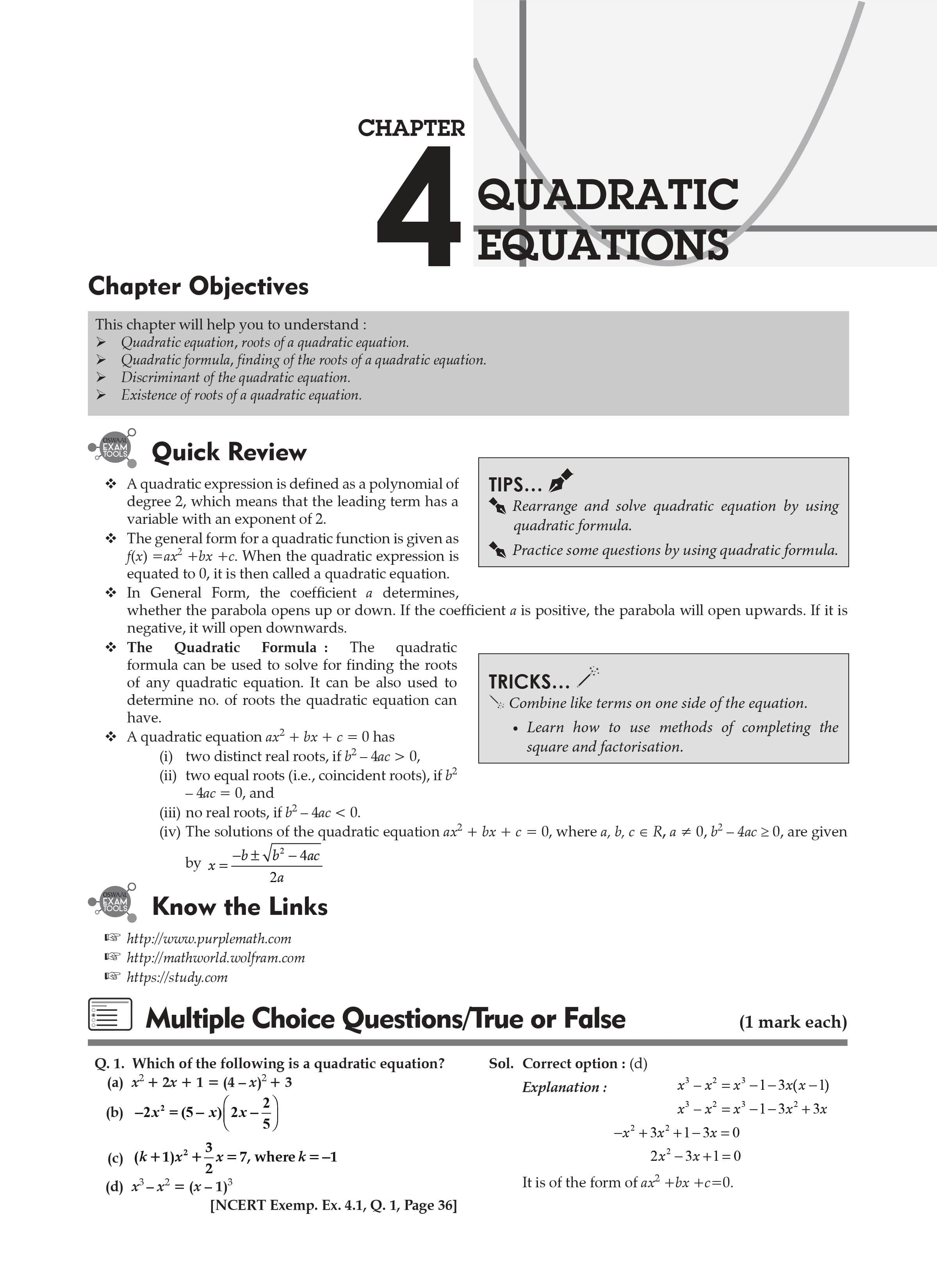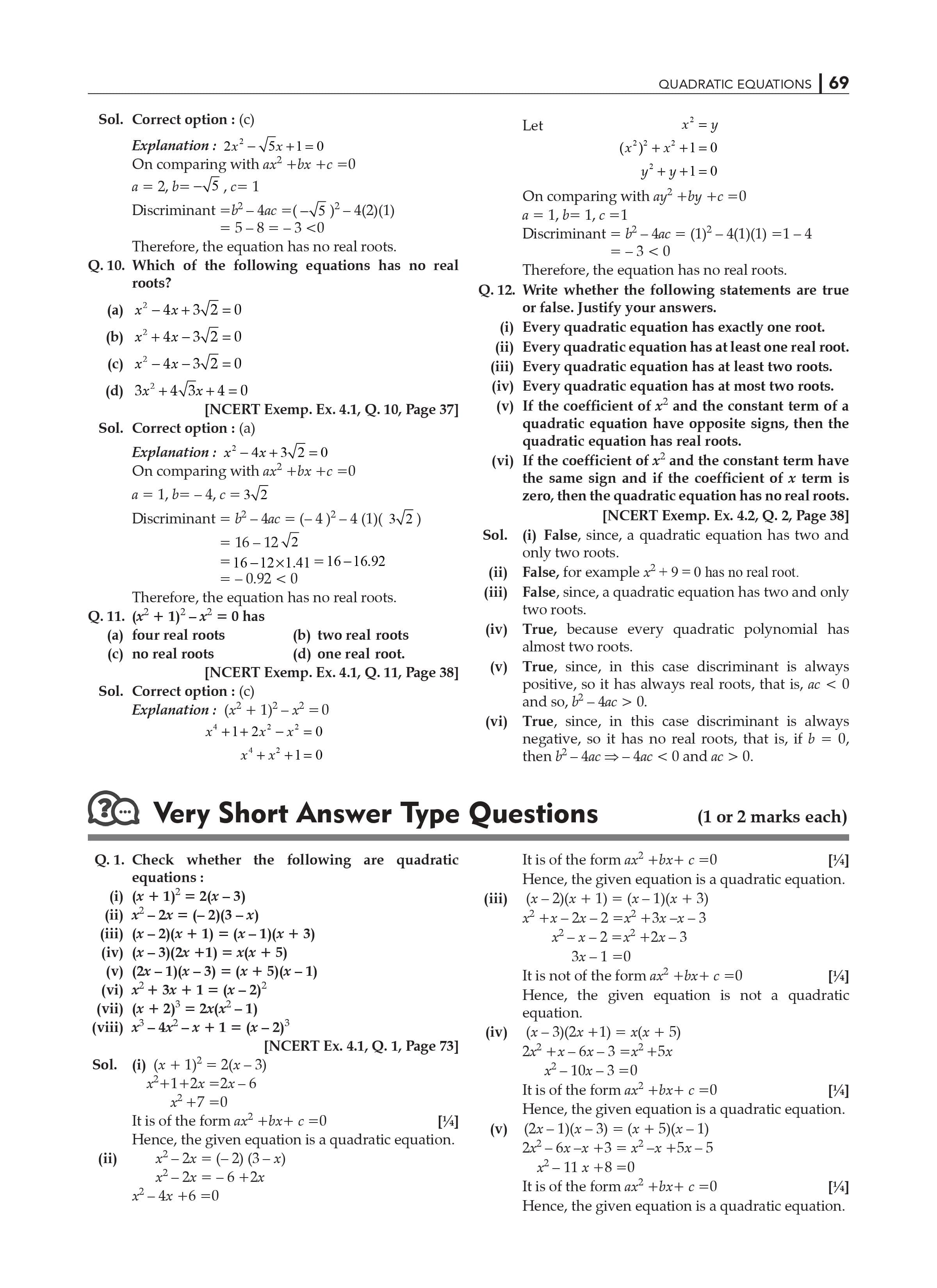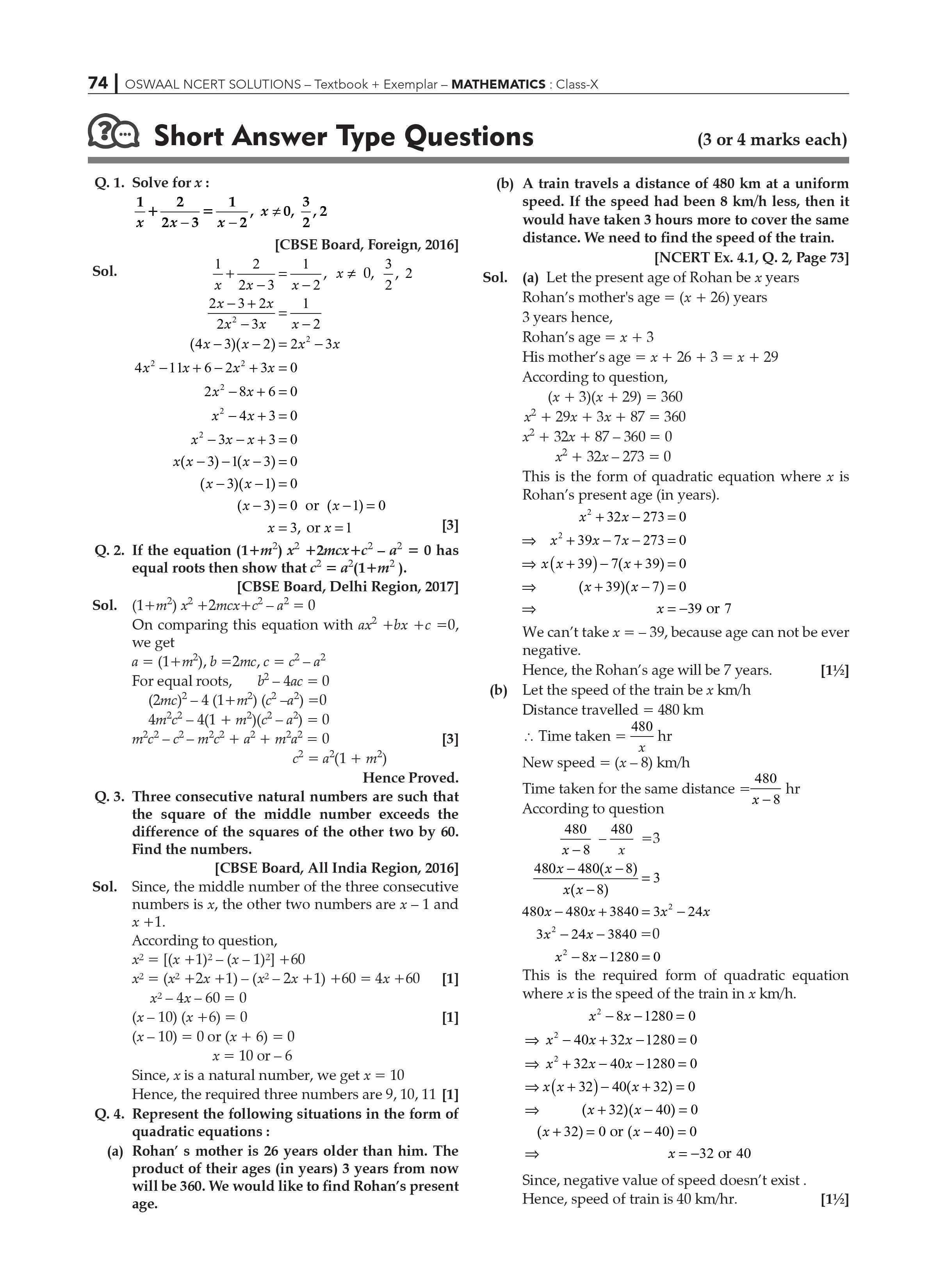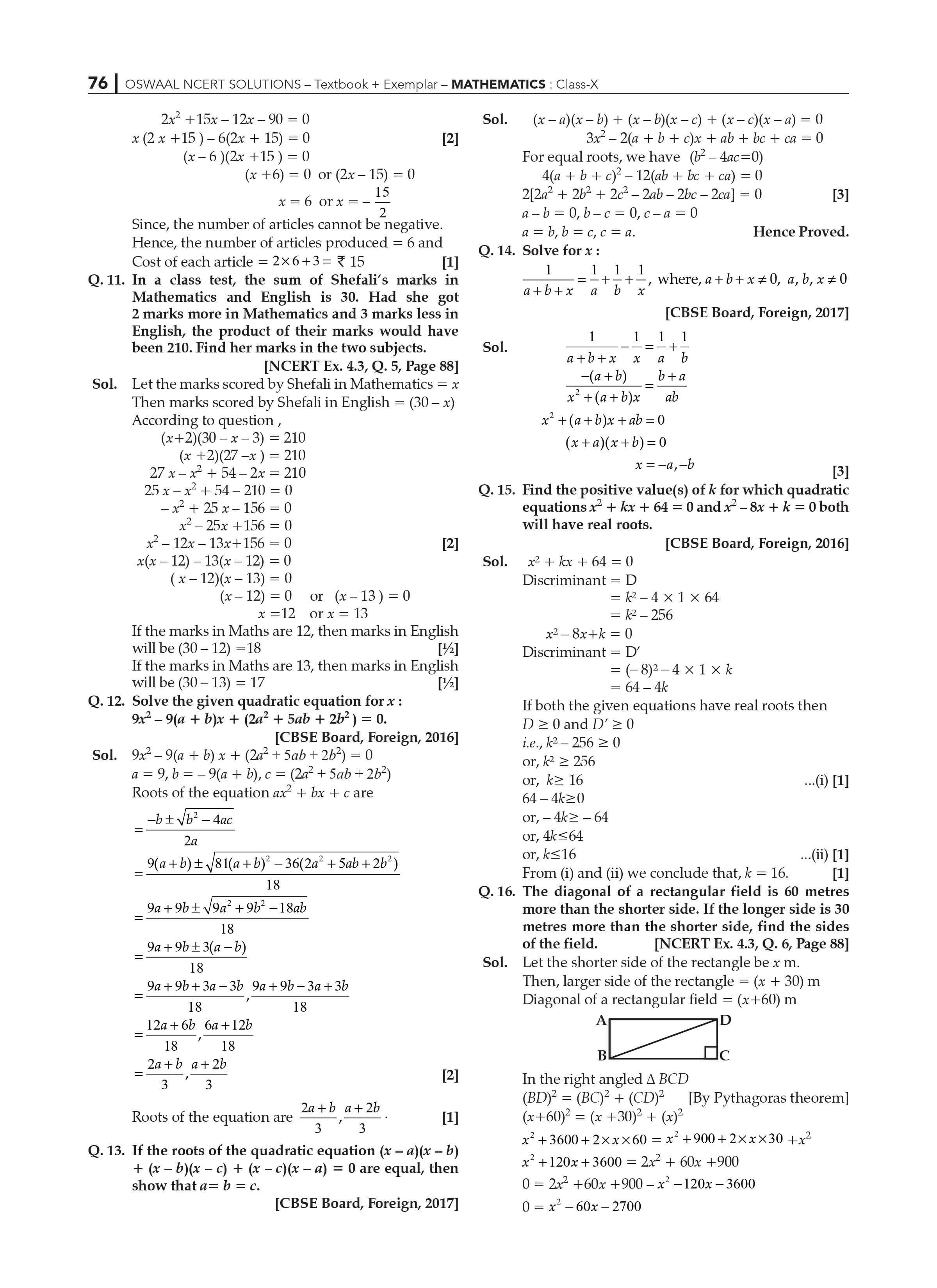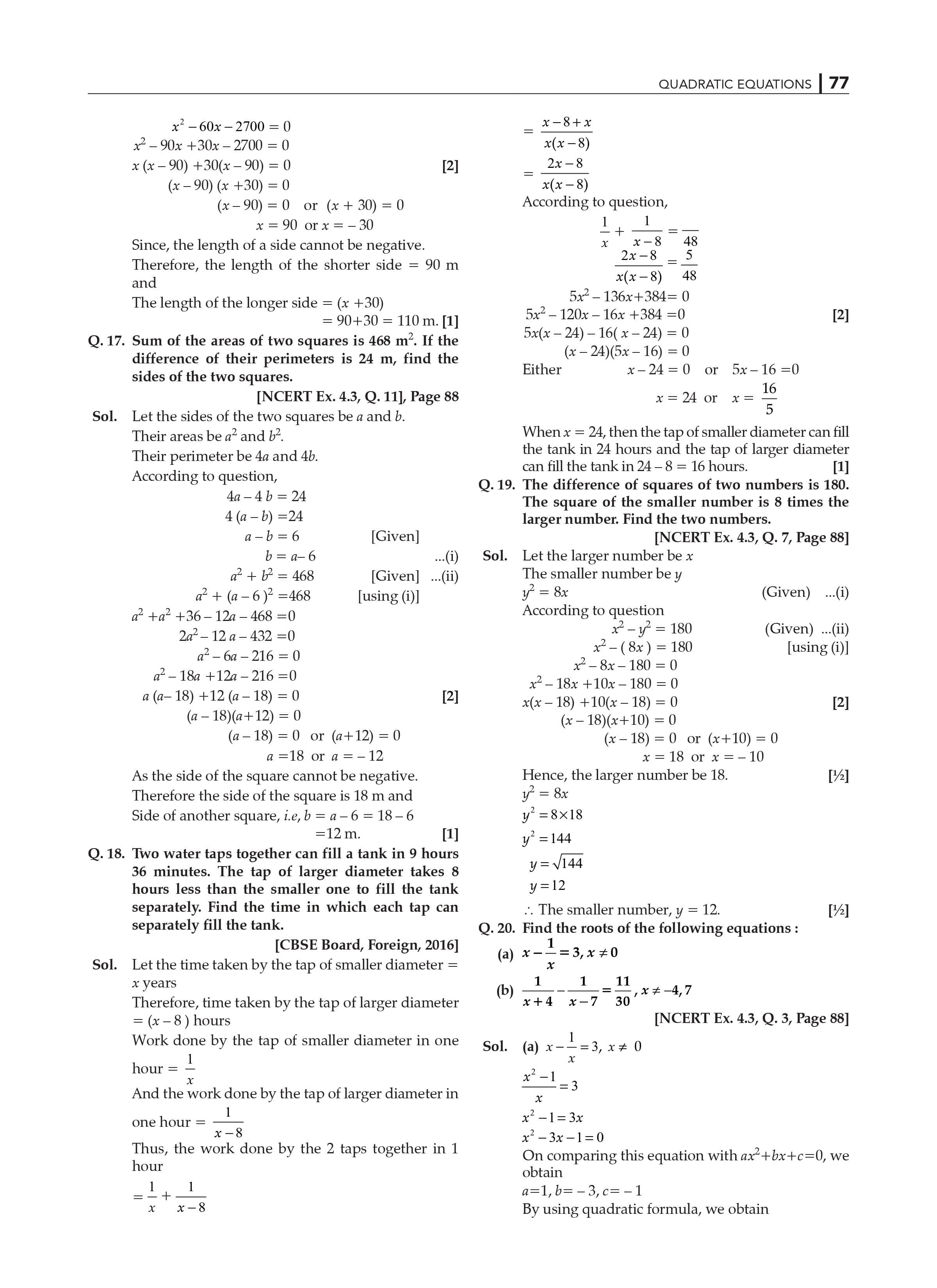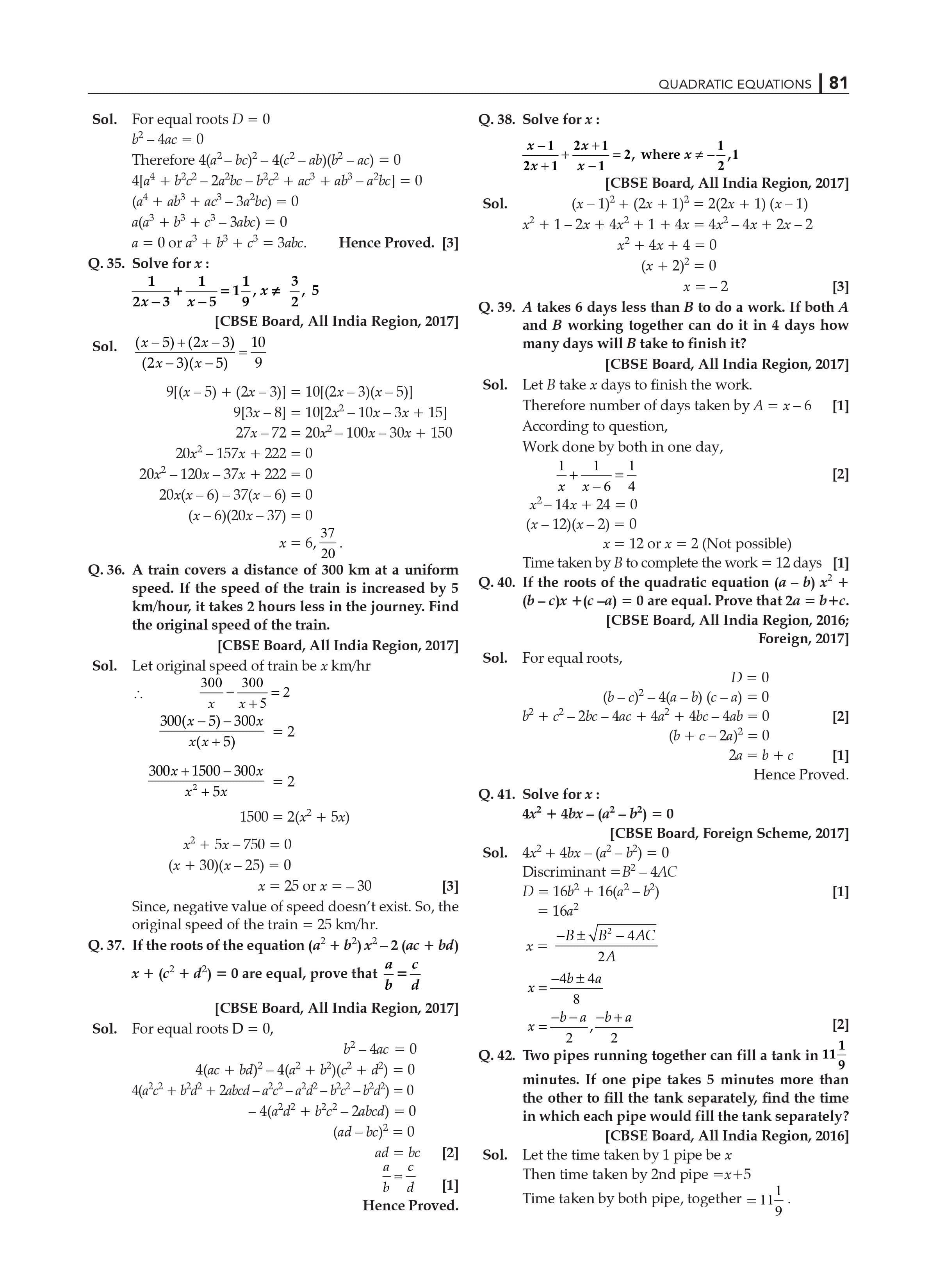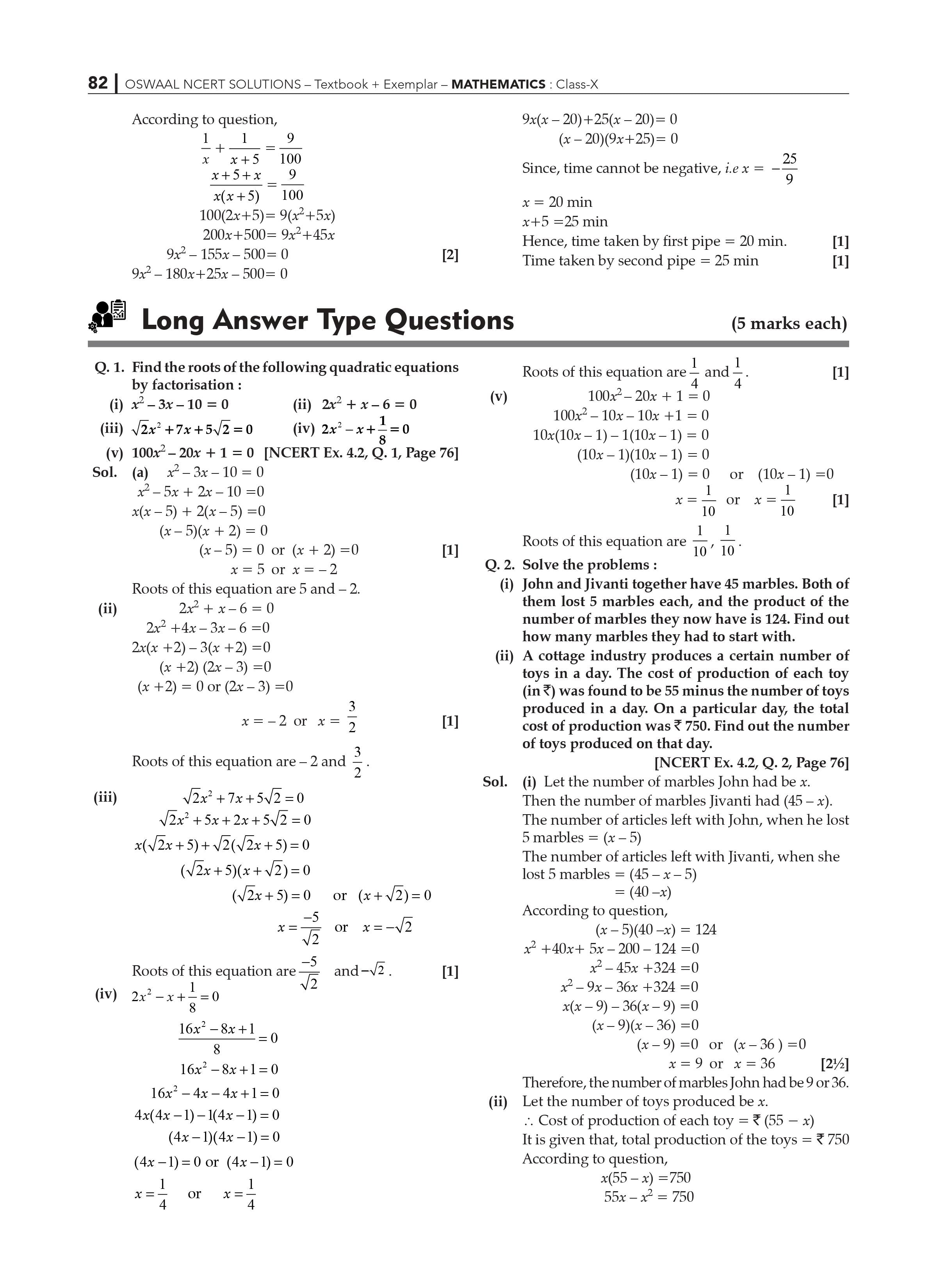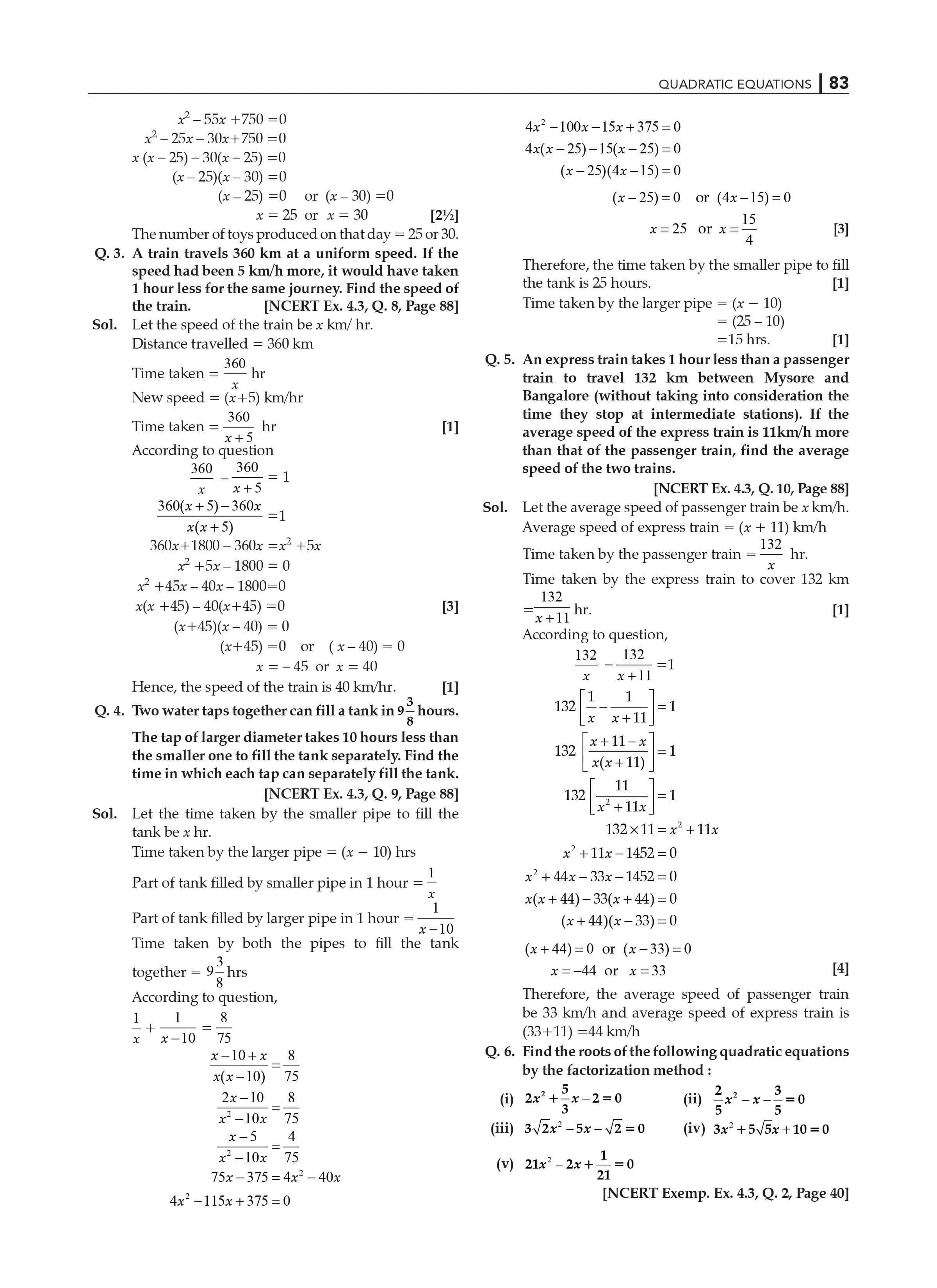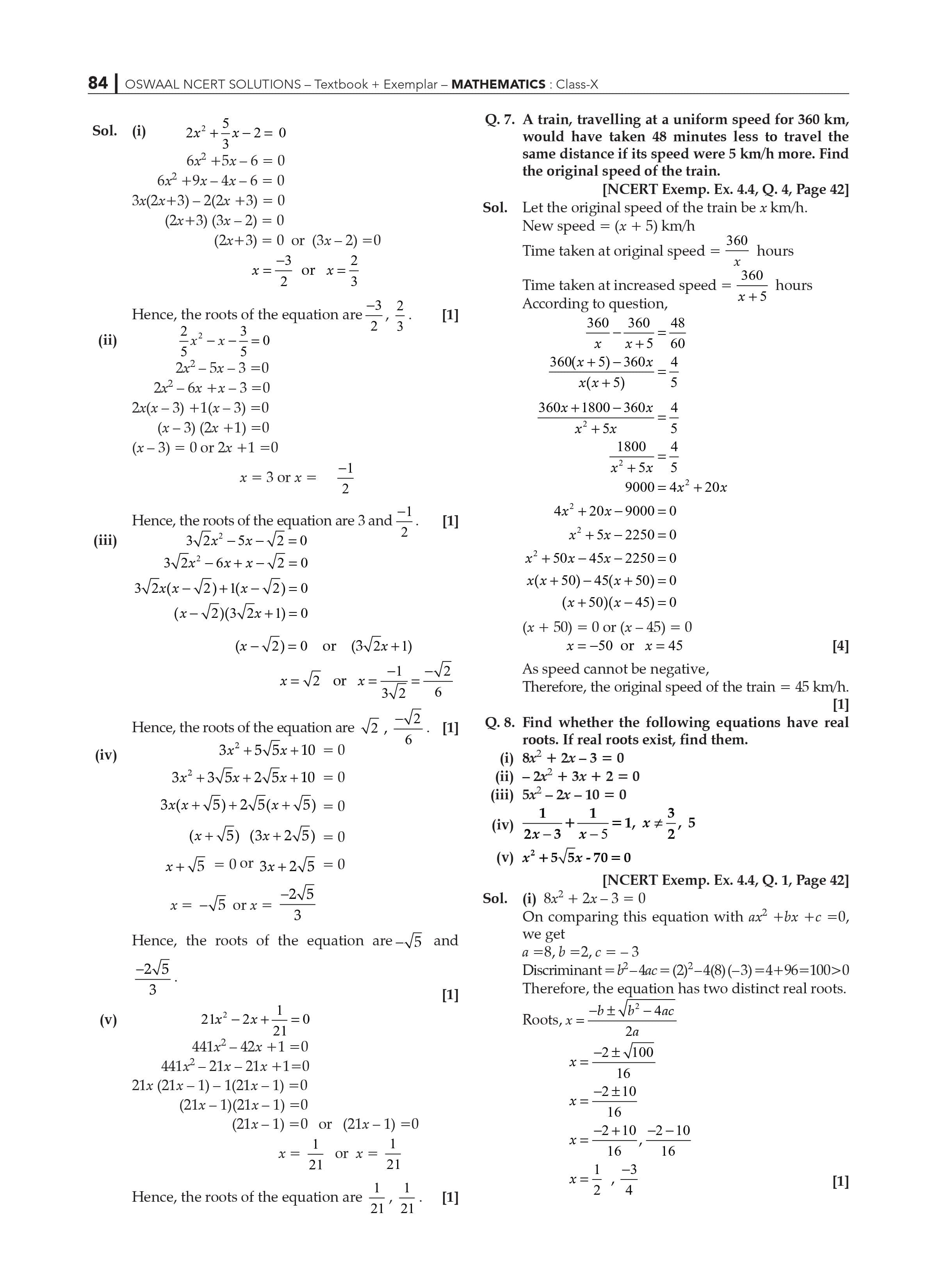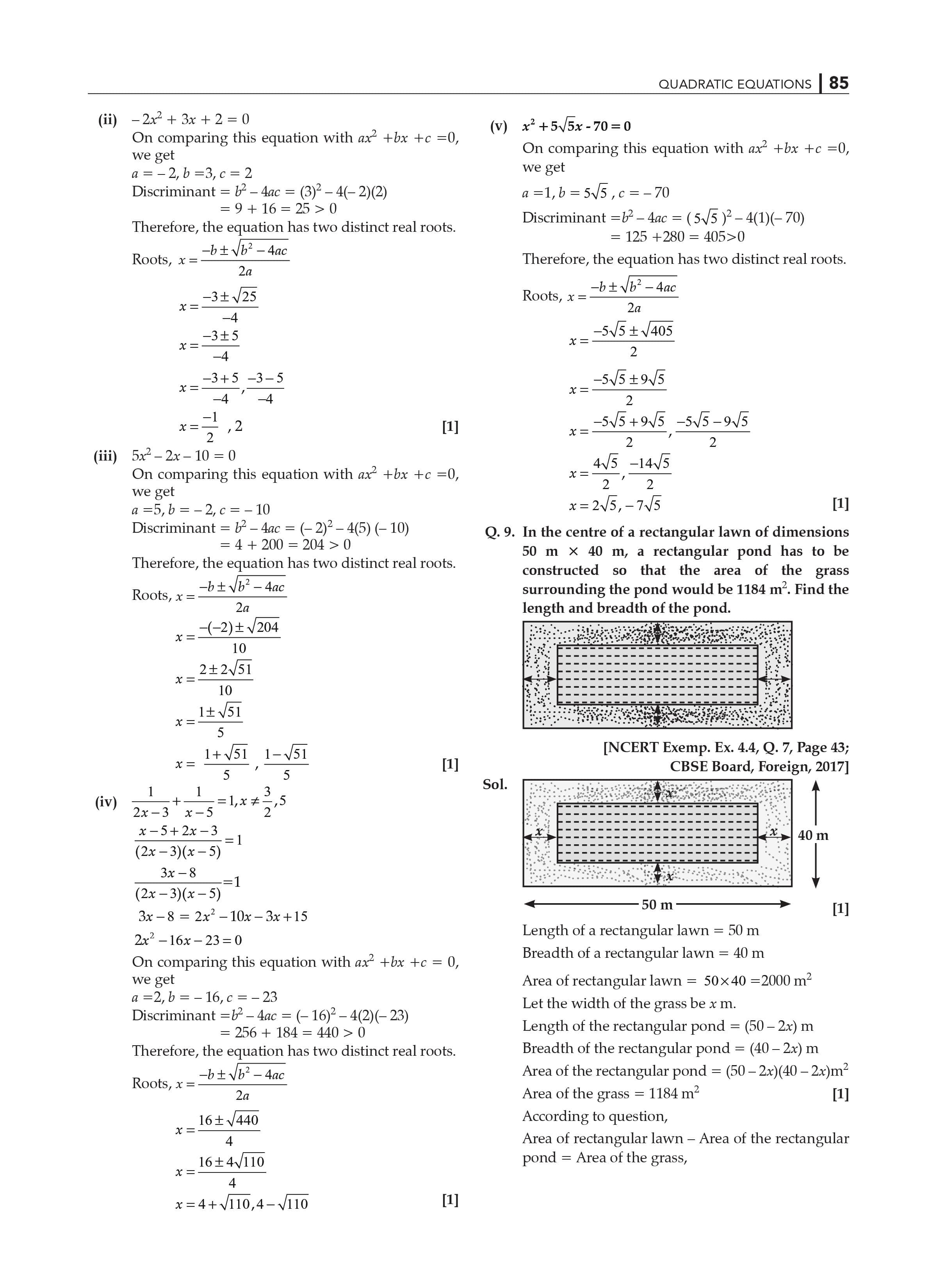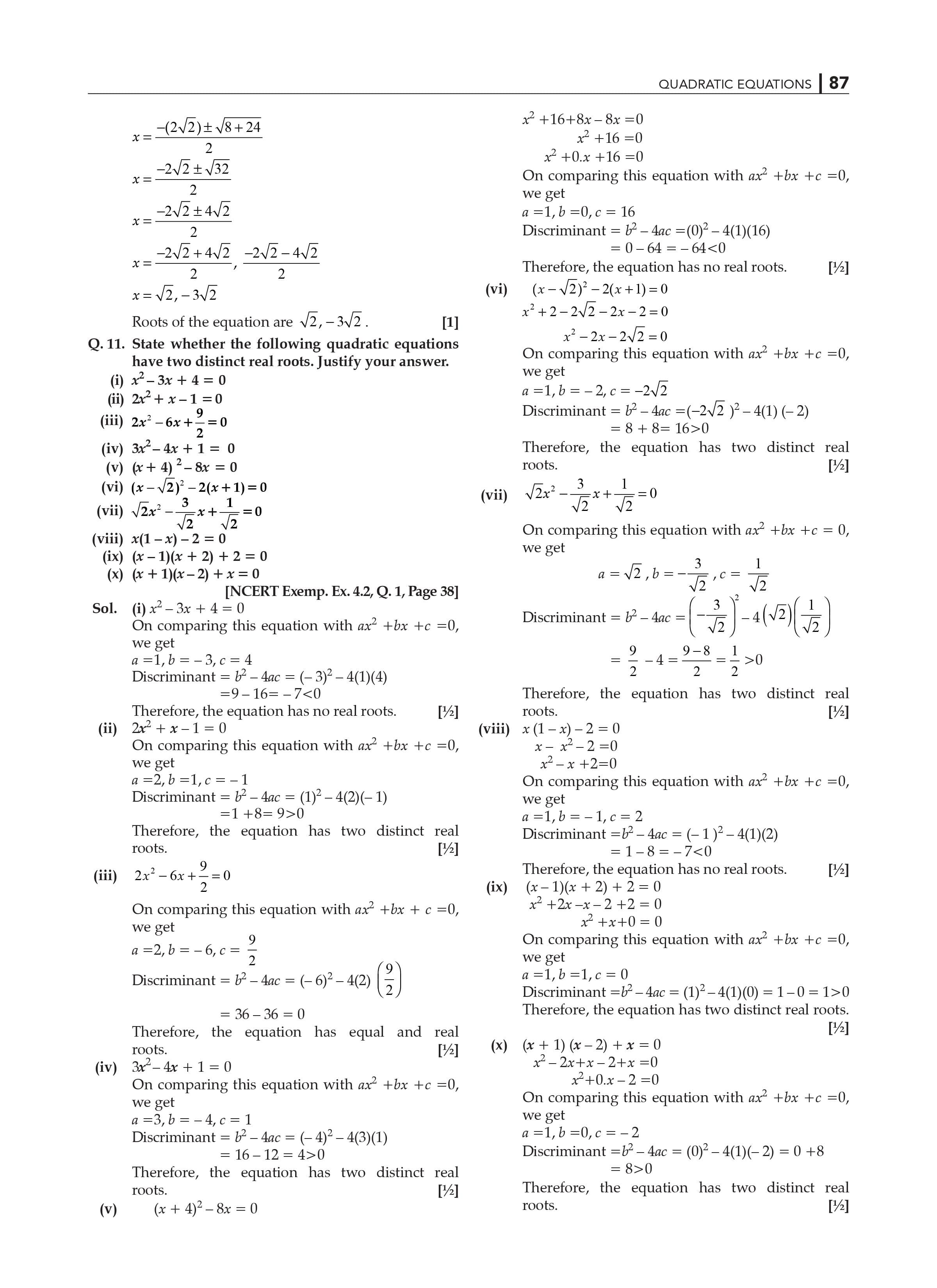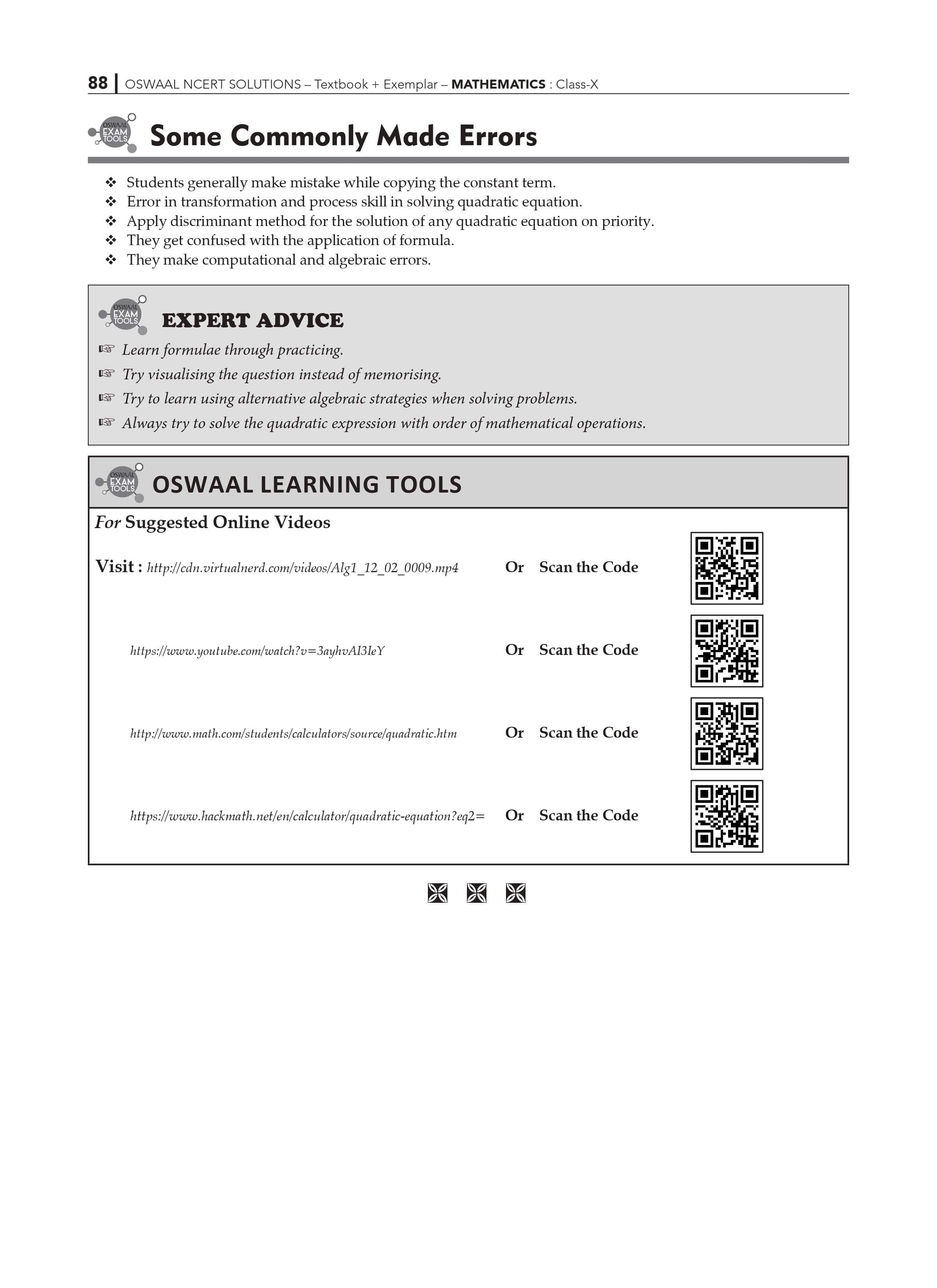Numerical NCERT solutions:

1. You have to represent the following given situations in the form of quadratic equations:
• The area of a rectangular piece of land is 628m2. The length of the given rectangular piece of land (in meters) is one more than twice its breadth. Help us find the length and breadth of the piece of the given land.

Solution: Let us consider,

Breadth of the rectangular plot = x m

Thus, the length of the plot = (2x + 1) m.

As we know,

Area of rectangle = length × breadth = 628 m2

Putting the value of length and breadth of the plot in the formula, we get,

(2x + 1) × x = 628

⇒ 2x2 + x =628

⇒ 2x2 + x – 628 = 0

Therefore, the length and breadth of plot, satisfies the quadratic equation, 2x2 + x – 628 = 0, which is the required representation of the problem mathematically.

• There are two integers given, the product of the given two consecutive positive integers is 408, find out what the two consecutive integers are.

Let us consider,

The first integer number = x

Thus, the next consecutive positive integer will be = x + 1

Product of two consecutive integers = x × (x +1) = 408

⇒ x2 + x = 408

⇒ x2 + x – 408 = 0

Therefore, the two integers x and x+1, satisfies the quadratic equation, x2 + x – 408 = 0, which is the required representation of the problem mathematically.

• A train is traveling at a uniform speed for a distance of 540 kilometers. Imagine if the train had been 9 km/hr less, then it would have taken?

Let us consider,

The speed of train = x km/h

And

Time taken to travel 540 km = 540/x km/hr.

As per second condition, the speed of train = (x – 9) km/h

Also given, the train will take 3 hours to cover the same distance.

Therefore, time taken to travel 540 km = (540/x) + 3 km/h

As we know,

Speed = Distance/Time

Therefore,

Distance = Time x Speed

(x – 9) {(540/x) + 3} = 540

⇒ 540 + 3x – 4860/x –27= 540

3x – 4860/x –27= 540-540

⇒ 3x – 4860/x = 27

⇒ x2 – 9x – 1620 = 0

Therefore, the speed of the train, satisfies the quadratic equation, x2 – 9x – 1620, which is the required representation of the problem mathematically.

NCERT Solutions for Class 10 Maths Exercise 4.1 of Chapter 4

Class 10 Mathematics NCERT Solutions Students will be learning how to crack mathematical problems which Involve this concept in Quadratic Equations. Exercise 4.1 of Chapter 4 is primarily concerned with the introduction of quadratic equations and their forms.

Mathematics is a very interesting subject that demands a tremendous amount of focus and understanding. Students preparing to take the first and second terms of the tenth grade could use the NCERT Solutions Class 10 as a practice guide because NCERT Solutions allow students to analyse themselves. After completing one chapter, students can solve the exercises and interpret their weak points in the chapter. This allows them to work on and improve their weaknesses before entering the examination hall.

There are approximately 7-8 marks questions

asked from this chapter and contribute a lot in gaining good marks if only the students practice maximum number of questions on a daily basis, analyse themselves, and keep working on their weaknesses till the exam comes.

NCERT Grade 10 Mathematics, Chapter 4 Quadratic Equations In the beginning, an example of a rectangular prayer hall is given, and the length and breadth of a rectangular hall are calculated based on the given situation. This example demonstrates how quadratic equations can be used to solve real-world problems.

Class 10 Maths NCERT Solutions Chapter 4- Quadratic Equations Exercise 4.1 contains all of the answers. The subject matter experts provided precise step-by-step answers to the questions.

The NCERT solutions for class 10 maths chapter 4 exercise 4.1 Quadratic Equations is the introduction of quadratic equations and their forms to the learners.

If there is an equation with the form a(x) = 0, a(x) being a polynomial of degree 2, then a(x)=0 is a quadratic equation; however, if the terms of a(x) are written in descending order of degrees, the standard form of the quadratic equation is obtained, which is represented by ax2 + bx + c = 0. The main goal of this activity is for students to be able to interpret a scenario in the form of a mathematical expression in order to solve it further.

In this exercise 4.1 of chapter 4, students will learn to convert the given equation into a standard form of quadratic equation using mathematical basic formulas and define the degree of the equation.

NCERT Solutions Class 10 Maths Chapter 4 Exercise 4.1 Quadratic Equations requires a solid understanding of algebra fundamentals. As a result, it is recommended that students review their previous polynomial concepts before beginning this chapter. They can start with the theory section and then move on to the solved examples.

NCERT solutions class 10 maths chapter 4 exercise 4.1 will require students to remember that a quadratic equation is one that can be simply represented in the given standard form. If this is not an option, the equation is not quadratic. A quadratic equation has the standard form ax2 + bx + c = 0. The majority of the problems in this exercise are concerned with representing a given equation in the standard form.

NCERT Solutions Class 10 Maths Chapter 4- Quadratic Equations Exercise 4.1 Highlights

• Subject matter experts provide the solutions.
• Every question in NCERT Solutions Class 10 Maths Chapter 4- Quadratic Equations Exercise 4.1 is thoroughly explained.
• The questions are written from the examination point of view.

Q1. In terms of exam preparation, what are the most important themes in NCERT Solutions for Class 10 Math Chapter 4?

1. NCERT Solutions Class 10 Maths Chapter 4 Quadratic Equations contains all of the solutions to the problems found in the NCERT textbook for Class 10 Maths for second term exam preparation. The concepts presented in NCERT Solutions for Class 10 Maths Chapter 4 Quadratic Equations are meaning and definition of quadratic equations, finding the roots of quadratic equations by factorization, finding the roots of quadratic equations by completing squares and nature of the roots. The questions from each section are carefully framed and answered by subject matter experts. NCERT Solutions for Class 10 is a detailed, step-by-step guide to all of the students' questions. If one wishes to do well in the examinations, the exercises in this chapter should be completed with the utmost sincerity.

Q2. What is the number of exercises in NCERT Solutions for Class 10 Maths Chapter 4?

1. There are four exercises in the fourth chapter of NCERT Solutions for Class 10 Maths. The first exercise deals with determining quadratic equations, the second with questions about finding the roots of quadratic equations by factorization, the third with questions about finding the roots of quadratic equations by completing squares, and the last with questions about the nature of the roots. By completing these exercises, students will be able to answer all of the quadratic equation-based questions.

Q3. Why are NCERT Solutions Class 10 Maths Chapter 4 important for getting good grades?

1. The NCERT solutions for class 10 maths chapter 4 were created by experts in their fields. They compiled the content into this textbook after extensive research, making it a valuable study resource. Quadratic equations have numerous applications in our daily lives; therefore, it is recommended that students thoroughly read this chapter in order to understand them. Furthermore, the CBSE board recommends that these solutions be implemented, making them critical.

Q4. Do I Need to Practice all Questions Given in Class 10 Maths NCERT Solutions Quadratic Equations?

1. The NCERT Solutions Class 10 Maths Quadratic Equations covers a wide range of topics that are important from the board exam's perspective. Problems based on topics such as the roots of a quadratic equation and the factorization method are often asked in exams. Hence, it is worthwhile to go through the entire theory and the solved examples from this chapter to get a better grasp of the concepts. We describe these ideas in great detail. As a result, the primary goal of these expert-formulated answers is to impart knowledge on the fundamental parts of math, allowing students to properly understand each concept.

NCERT Solutions for Class 10 Maths Exercise 4.2 of Chapter 4

Class 10 Mathematics NCERT Solutions Students will be learning how to crack mathematical problems which Involve this concept in Quadratic Equations. Exercise 4.2 of Chapter 4 helps students understand how to solve the quadratic equations using the Factorization method.

Mathematics is a very interesting subject that demands a tremendous amount of focus and understanding. After completing one chapter, students can solve the exercises and interpret their weak points in the chapter. This allows them to work on and improve their weaknesses before entering the examination hall.

There are approximately 7-8 marks questions

asked from this chapter and contribute a lot in gaining good marks if only the students practice maximum number of questions on a daily basis, analyse themselves, and keep working on their weaknesses till the exam comes.

NCERT Solutions Class 10 Maths Chapter 4 Exercise 4.2 Quadratic Equations requires a solid understanding of algebra fundamentals. The students who have not completely understood the concept of Algebra will find it difficult to understand and will face hardship while solving the equations.  As a result, it is recommended that students review their previous polynomial concepts before beginning this chapter. They can start with the theory section and then move on to the solved examples.

NCERT solutions for Chapter 4 Exercise 4.2 in Class 10 Maths Quadratic Equations explains how to solve quadratic equations using factorization, the concept of roots, and the zero of a polynomial. If a number is the root of a quadratic equation, it is also the zero of a polynomial. Students should be familiar with factoring quadratic polynomials by splitting their middle terms. As a result, they will be able to find the roots using the same method, namely factoring the quadratic equation into two linear factors and then equating each factor to zero.

It's important to remember that any quadratic polynomial has two zeroes, so the quadratic equation has at least two roots.

The exercise 4.2 of chapter 4 consists of six short questions in which students must solve quadratic equations using the factorization method.

NCERT solutions class 10 maths chapter 4 exercise 4.2 Quadratic Equations necessitates that student comprehend the significance of solving problems in a step-by-step manner in order to avoid confusion. As a result, the students are advised to closely follow the solved examples in order to gain an understanding of how to approach the questions logically, using proper statements and the correct explanation.

The NCERT solutions for class 10 maths chapter 4 exercise 4.2 provide a detailed step-by-step solution to all six questions using the factorization method. Students must ensure that they verify the roots when answering the exercise questions in order to cross-check their answers. Because this is a critical topic, students should go over all of the questions at least twice.

Math in 10th grade RD Sharma solves a number of questions based on the concept of Quadratic equations NCERT Class 10 Math Solutions

NCERT Solutions Class 10 Maths Chapter 4- Quadratic Equations Exercise 4.2 Highlights

• Subject matter experts provide the solutions.
• Every question in NCERT Solutions Class 10 Maths Chapter 4- Quadratic Equations Exercise 4.2 is thoroughly explained.
• The questions are written from the examination point of view.

Q1. In terms of exam preparation, what are the most important themes in NCERT Solutions for Class 10 Math Chapter 4?

1. NCERT Solutions Class 10 Maths Chapter 4 Quadratic Equations contains all of the solutions to the problems found in the NCERT textbook for Class 10 Maths for 2023 exam preparation. The concepts presented in NCERT Solutions for Class 10 Maths Chapter 4 Quadratic Equations are significance and definition of quadratic equations, concepts involving finding the roots of quadratic equations by factorization, finding the roots of quadratic equations by completing squares and nature of the roots. The questions from each section are carefully framed and answered by subject matter experts. NCERT Solutions for Class 10 is a detailed, step-by-step guide to all of the students' questions. If one wishes to do well in the examinations, the exercises in this chapter should be completed with the utmost sincerity.

Q2. What is the number of exercises in NCERT Solutions for Class 10 Maths Chapter 4?

1. There are four exercises in the fourth chapter of NCERT Solutions for Class 10 Maths. The first exercise deals with determining quadratic equations, the second with questions about finding the roots of quadratic equations by factorization, the third with questions about finding the roots of quadratic equations by completing squares, and the last with questions about the nature of the roots. By completing these exercises, students will be able to answer all of the quadratic equation-based questions.

Q3.  Why are NCERT Solutions Class 10 Maths Chapter 4 important for getting good grades?

1. The NCERT solutions for class 10 maths chapter 4 were created by experts in their fields. They compiled the content into this textbook after extensive research, making it a valuable study resource. Quadratic equations have numerous applications in our daily lives; therefore, it is recommended that students thoroughly read this chapter in order to understand them. Furthermore, the CBSE board recommends that these solutions be implemented, making them critical.

Q4. Do I Need to Practice all Questions Given in Class 10 Maths NCERT Solutions Quadratic Equations?

1. The NCERT Solutions Class 10 Maths Quadratic Equations covers a wide range of topics that are important from the board exam's perspective. Problems based on topics such as the roots of a quadratic equation and the factorization method are often asked in exams. Hence, it is worthwhile to go through the entire theory and the solved examples from this chapter to get a better grasp of the concepts. We describe these ideas in great detail. As a result, the primary goal of these expert-formulated answers is to impart knowledge on the fundamental parts of math, allowing students to properly understand each concept.

Q5. How do I get started with Chapter 4 of NCERT Solutions for Class 10 Math?

1. To begin, use the website to learn and practise.

understand the meaning of linear equations Then, on the website, review the responses provided by their professionals. Students will gain a thorough understanding of all of the concepts covered in Chapter 4 of the NCERT Solutions for Class 10 Math by completing these activities.

NCERT Solutions for Class 10 Maths Exercise 4.2 of Chapter 4

Class 10 Mathematics NCERT Solutions Students will be learning how to crack mathematical problems which Involve this concept in Quadratic Equations. Exercise 4.2 of Chapter 4 helps students understand how to solve the quadratic equations using the Factorization method.

Mathematics is a very interesting subject that demands a tremendous amount of focus and understanding. After completing one chapter, students can solve the exercises and interpret their weak points in the chapter. This allows them to work on and improve their weaknesses before entering the examination hall.

There are approximately 7-8 marks questions

asked from this chapter and contribute a lot in gaining good marks if only the students practice maximum number of questions on a daily basis, analyse themselves, and keep working on their weaknesses till the exam comes.

NCERT Solutions Class 10 Maths Chapter 4 Exercise 4.2 Quadratic Equations requires a solid understanding of algebra fundamentals. The students who have not completely understood the concept of Algebra will find it difficult to understand and will face hardship while solving the equations.  As a result, it is recommended that students review their previous polynomial concepts before beginning this chapter. They can start with the theory section and then move on to the solved examples.

NCERT solutions for Chapter 4 Exercise 4.2 in Class 10 Maths Quadratic Equations explains how to solve quadratic equations using factorization, the concept of roots, and the zero of a polynomials. If a number is the root of a quadratic equation, it is also the zero of a polynomials. Students should be familiar with factoring quadratic polynomials by splitting their middle terms. As a result, they will be able to find the roots using the same method, namely factoring the quadratic equation into two linear factors and then equating each factor to zero.

It's important to remember that any quadratic polynomial has two zeroes, so the quadratic equation has at least two roots.

The exercise 4.2 of chapter 4 consists of six short questions in which students must solve quadratic equations using the factorization method.

NCERT solutions class 10 maths chapter 4 exercise 4.2 Quadratic Equations necessitates that student comprehend the significance of solving problems in a step-by-step manner in order to avoid confusion. As a result, the students are advised to closely follow the solved examples in order to gain an understanding of how to approach the questions logically, using proper statements and the correct explanation.

The NCERT solutions for class 10 maths chapter 4 exercise 4.2 provide a detailed step-by-step solution to all six questions using the factorization method. Students must ensure that they verify the roots when answering the exercise questions in order to cross-check their answers. Because this is a critical topic, students should go over all of the questions at least twice.

Math in 10th grade RD Sharma solves a number of question based on the concept of Quadratic equations NCERT Class 10 Math Solutions

NCERT Solutions Class 10 Maths Chapter 4- Quadratic Equations Exercise 4.2 Highlights

• Subject matter experts provide the solutions.
• Every question in NCERT Solutions Class 10 Maths Chapter 4- Quadratic Equations Exercise 4.2 is thoroughly explained.
• The questions are written from the examination point of view.

Q1. In terms of exam preparation, what are the most important themes in NCERT Solutions for Class 10 Math Chapter 4?

1. NCERT Solutions Class 10 Maths Chapter 4 Quadratic Equations contains all of the solutions to the problems found in the NCERT textbook for Class 10 Maths for 2023 exam preparation. The concepts presented in NCERT Solutions for Class 10 Maths Chapter 4 Quadratic Equations are significance and definition of quadratic equations, concepts involving finding the roots of quadratic equations by factorization, finding the roots of quadratic equations by completing squares and nature of the roots. The questions from each section are carefully framed and answered by subject matter experts. NCERT Solutions for Class 10 is a detailed, step-by-step guide to all of the students' questions. If one wishes to do well in the examinations, the exercises in this chapter should be completed with the utmost sincerity.

Q2. What is the number of exercises in NCERT Solutions for Class 10 Maths Chapter 4?

1. There are four exercises in the fourth chapter of NCERT Solutions for Class 10 Maths. The first exercise deals with determining quadratic equations, the second with questions about finding the roots of quadratic equations by factorization, the third with questions about finding the roots of quadratic equations by completing squares, and the last with questions about the nature of the roots. By completing these exercises, students will be able to answer all of the quadratic equation-based questions.

Q3.  Why are NCERT Solutions Class 10 Maths Chapter 4 important for getting good grades?

1. The NCERT solutions for class 10 maths chapter 4 were created by experts in their fields. They compiled the content into this textbook after extensive research, making it a valuable study resource. Quadratic equations have numerous applications in our daily lives; therefore, it is recommended that students thoroughly read this chapter in order to understand them. Furthermore, the CBSE board recommends that these solutions be implemented, making them critical.

Q4. Do I Need to Practice all Questions Given in Class 10 Maths NCERT Solutions Quadratic Equations?

1. The NCERT Solutions Class 10 Maths Quadratic Equations covers a wide range of topics that are important from the board exam's perspective. Problems based on topics such as the roots of a quadratic equation and the factorization method are often asked in exams. Hence, it is worthwhile to go through the entire theory and the solved examples from this chapter to get a better grasp of the concepts. We describe these ideas in great detail. As a result, the primary goal of these expert-formulated answers is to impart knowledge on the fundamental parts of math, allowing students to properly understand each concept.

Q5. How do I get started with Chapter 4 of NCERT Solutions for Class 10 Math?

1. To begin, use the website to learn and practise.

understand the meaning of linear equations Then, on the website, review the responses provided by their professionals. Students will gain a thorough understanding of all of the concepts covered in Chapter 4 of the NCERT Solutions for Class 10 Math by completing these activities.

NCERT Solutions for Class 10 Maths Exercise 4.3 of Chapter 4

Class 10 Mathematics NCERT Solutions Students will be learning how to crack mathematical problems which Involve this concept in Quadratic Equations. Exercise 4.3 of Chapter 4 helps students understand how to solve the quadratic equations by completing a square and using the quadratic formula to solve a quadratic equation.

Mathematics is a very interesting subject that demands a tremendous amount of focus and understanding. Students preparing to take the first and second terms of the tenth grade could use the NCERT Solutions Class 10 as a practice guide because NCERT Solutions allow students to analyse themselves. After completing one chapter, students can solve the exercises and interpret their weak points in the chapter. This allows them to work on and improve their weaknesses before entering the examination hall.

There are approximately 7-8 marks questions asked from this chapter and contribute a lot in gaining good marks if only the students practice maximum number of questions on a daily basis, analyse themselves, and keep working on their weaknesses till the exam comes.

NCERT Solutions Class 10 Maths Chapter 4 Exercise 4.3 Quadratic Equations requires a solid understanding of algebra fundamentals. The students who have not completely understood the concept of Algebra will find it difficult to understand and will face hardship while solving the equations.  As a result, it is recommended that students review their previous polynomial concepts before beginning this chapter. They can start with the theory section and then move on to the solved examples.

NCERT solutions for class 10 maths chapter 4 exercise 4.3 Quadratic Equations will help the students ace the concepts of solving a quadratic equation by completing a square and applying the quadratic formula to derive the roots of a quadratic equation. If any quadratic equation can be converted to the form of (x + a)2 – b2= 0, then it becomes easy to find its roots. This is known as the method of completing the square.

The students must also practice learning the formula to find the roots of the equation ax2 + bx + c = 0 which is:

x = [-b ± √ (b2 - 4ac)] / 2a

NCERT solutions for chapter 4 exercise 4.3 in class 10 maths Quadratic Equations will teach students how to solve quadratic equations by completing a square and using the quadratic formula to find the roots of a quadratic equation. It is simple to find the roots of any quadratic equation that can be converted to the form (x + a)2 – b2 = 0. This method of solving quadratic equations is referred to as the square-completion method.

Students must also practise learning the formula for finding the roots of the equation ax2 + bx + c = 0, which is as follows:

x= [-b + √b2 - 4ac] / 2a

Students will learn how to apply the quadratic formula with the help of this NCERT solutions class 10 maths chapter 4 exercise 4.3 and how it can be further analysed to draw logical conclusions. In addition, they learn how to convert a mathematical equation into a square and identify the nature of the roots. There are 11 questions based on these concepts in this exercise.

NCERT solutions for class ten maths chapter four exercise 4.3 Quadratic Equations allows students to compare two methods for finding the roots of a quadratic equation. The first is to finish the squares, and the second is to use the quadratic formula. To move through the exercise questions smoothly, students should be thoroughly familiar with the concept and calculations involving square roots.

NCERT solutions for chapter 4 exercise 4.3 in class 10 maths Apart from remembering the quadratic formula, Quadratic Equations outlines certain important conditions for obtaining the roots; for example, if the value of b2 – 4ac is 0, the roots of the quadratic equation: ax2 + bx + c = 0 are given by [-b (b2+ 4ac)]/ 2a

It is reasonable to use the quadratic formula.

one of the quickest methods for finding the roots of a quadratic equation Referring to examples can be extremely beneficial in understanding this method. Furthermore, if not specified in the questions, children should aim to solve the problem using this technique.

NCERT Solutions Class 10 Maths Chapter 4- Quadratic Equations Exercise 4.3 Highlights

• Subject matter experts provide the solutions.
• Every question in NCERT Solutions Class 10 Maths Chapter 4- Quadratic Equations Exercise 4.3 is thoroughly explained.
• The questions are written from the examination point of view.

Q1. In terms of exam preparation, what are the most important themes in NCERT Solutions for Class 10 Math Chapter 4?

1. NCERT Solutions Class 10 Maths Chapter 4 Quadratic Equations contains all of the solutions to the problems found in the NCERT textbook for Class 10 Maths for second term exam preparation. The concepts presented in NCERT Solutions for Class 10 Maths Chapter 4 Quadratic Equations are significance and definition of quadratic equations, concepts involving finding the roots of quadratic equations by factorization, finding the roots of quadratic equations by completing squares and nature of the roots.The questions from each section are carefully framed and answered by subject matter experts. NCERT Solutions for Class 10 is a detailed, step-by-step guide to all of the students' questions. If one wishes to do well in the examinations, the exercises in this chapter should be completed with the utmost sincerity.

Q2. What is the number of exercises in NCERT Solutions for Class 10 Maths Chapter 4?

1. There are four exercises in the fourth chapter of NCERT Solutions for Class 10 Maths. The first exercise deals with determining quadratic equations, the second with questions about finding the roots of quadratic equations by factorization, the third with questions about finding the roots of quadratic equations by completing squares, and the last with questions about the nature of the roots. By completing these exercises, students will be able to answer all of the quadratic equation-based questions.

Q3. Why are NCERT Solutions Class 10 Maths Chapter 4 important for getting good grades?

1. The NCERT solutions for class 10 maths chapter 4 were created by experts in their fields. They compiled the content into this textbook after extensive research, making it a valuable study resource. Quadratic equations have numerous applications in our daily lives; therefore, it is recommended that students thoroughly read this chapter in order to understand them. Furthermore, the CBSE board recommends that these solutions be implemented, making them critical.

Q4. Do I Need to Practice all Questions Given in Class 10 Maths NCERT Solutions Quadratic Equations?

1. The NCERT Solutions Class 10 Maths Quadratic Equations covers a wide range of topics that are important from the board exam's perspective. Problems based on topics such as the roots of a quadratic equation and the factorization method are often asked in exams. Hence, it is worthwhile to go through the entire theory and the solved examples from this chapter to get a better grasp of the concepts. We describe these ideas in great detail. As a result, the primary goal of these expert-formulated answers is to impart knowledge on the fundamental parts of math, allowing students to properly understand each concept.

Q5. How do I get started with Chapter 4 of NCERT Solutions for Class 10 Math?

1. To begin, use the website to learn and practise.

understand the meaning of linear equations Then, on the website, review the responses provided by their professionals. Students will gain a thorough understanding of all of the concepts covered in Chapter 4 of the NCERT Solutions for Class 10 Math by completing these activities.

Key features of the NCERT solutions for Class 10th chapter 4 Quadratic Equations:

1. The highly trained faculty have designed all the solutions with utmost precision and simple to understand text.
2. All the responses to the questions are entirely correct, hence can be used by the students preparing for the CBSE board exams.
3. All the NCERT solutions to all the questions available in the textbook can be easily found here.
4. The exercise questions that are present in the NCERT textbook are all answered in step-to-step manner hence allowing the students to not only attain a good score just on the final answer but also on each and every step.

For more such questions to practice, students can refer to all the other study materials which are offered at CBSE Books Class 10.

Frequently asked questions on NCERT solutions for class 10th chapter 4 Quadratic Equations:

1. Mention all the important concepts that you learn in NCERT solutions for class 10 math chapter 4 Quadratic equations?

All the concepts presented in NCERT solutions for class 10 math chapter 4 Quadratic Equations are the meaning and definitions of quadratic equations, finding the different types of roots of the equations by factorization or by finding the roots of the quadratic equations by completing the squares and nature of the given roots.

1. Is OSWAAL’s website really providing all the answers for NCERT solutions chapter 4 Quadratic Equations?

Yes, you can avail all the PDFs of NCERT solutions for class 10 Quadratic Equations. All the solutions are provided by the expert and best in their respective fields. Those who wish to score good marks are advised to solve the NCERT textbook.

3. How many exercises are there in the NCERT solutions for class      10 chapter 4?

The Fourth Chapter of NCERT solutions for class 10 has in total 4 exercises.

The first exercise contains the topic of determining the quadratic equations, the second exercise deals with the questions of finding the roots of the given quadratic equations by factorization, the third exercise has questions that require finding the roots of quadratic equations by completing squares and the final exercise deals with the questions that are based on the nature of the given roots in the question. By solving all these exercises, the students are able to answer all the questions that can be formed using the quadratic equations. Hence acing this chapter completely and being fully prepared for the board exams.